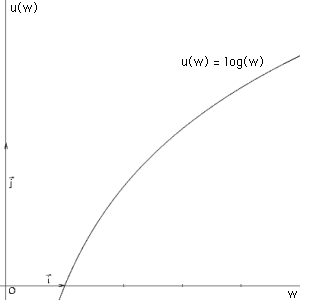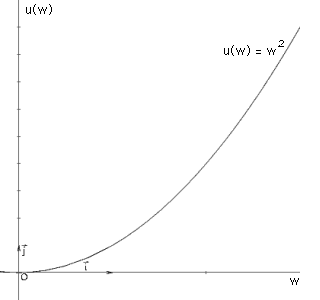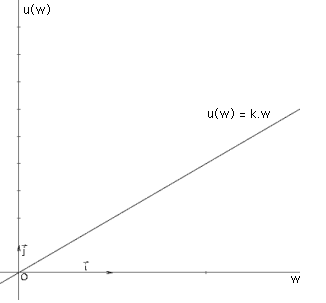Collapse Sidebar

 Students / SubjectsHandbook > Decision-Making Under Uncertainty > Basic Concepts > Section 4: An Introduction to Risk-AversionPrinter Friendly

Decision-Making Under Uncertainty - Basic Concepts

An Introduction to Risk-Aversion

In the previous section, we introduced the concept of an expected utility function, and stated how people maximize their expected utility when faced with a decision involving outcomes with known probabilities. So an expected utility function over a gamble g takes the form:

u(g) = p1u(a1) + p2u(a2) + ... + pnu(an)

where the utility function over the outcomes, i.e. u(ai), is the Bernoulli utility function.

In Bernoulli's formulation, this function was a logarithmic function, which is strictly concave, so that the decision-maker's expected utility from a gamble was less than its expected value. Bernoulli assumed this would hold, since most people are risk-averse - they prefer a more certain outcome to a less certain one. This does seem like a reasonable assumption at first glance.

But is this always true? Most of us buy insurance - for cars, homes, and pretty much anything we consider valuable. The rationale is straightforward - even though the probability of losing or damaging the item insured may be small, the potential loss would be so huge, that most people would rather pay moderate amounts of money for certain, than lose a large sum with a very small probability.

But there's another side to this - the insurance companies. If it's logical for people to buy insurance, how come it's also logical to sell it? After all, insurance companies are in the business to make a profit. If we as individuals are better off paying comparatively small, fixed amounts at regular intervals (as an insurance premium) than risking a large loss, how is the insurance company better off by accepting these small amounts, and agreeing to risk a large loss? If buyers of insurance are indeed risk-averse - what about those who sell it?

Obviously Bernoulli's assumption does not always hold. Using the von Neumann-Morgenstern framework, we can define people's attitudes towards risk much more precisely, without making any prior assumptions about their behavior.

Different Risk Attitudes

We can group people's attitudes towards risk into 3 distinct categories, based on the form of their respctive Bernoulli utility functions. Let's illustrate this with a simple gamble based on a coin toss, which pays \$10 if the coin lands heads, and \$20 if the coin lands tails. The expected value of this gamble is, of course: (0.5 * 10) + (0.5 * 20) = \$15.

1. Risk-Averse: If a person's utility of the expected value of a gamble is greater than their expected utility from the gamble itself, they are said to be risk-averse. This is a more precise definition of Bernoulli's idea.
Risk-averse behavior is captured by a concave Bernoulli utility function, like a logarithmic function. For the above gamble, a risk-averse person whose Bernoulli utility function took the form u(w) = log(w), where w was the outcome, would have an expected utility over the gamble of:
0.5 * log(10) + 0.5 * log(20) = 1.15,
while their utility of the expected value of the gamble is log(15) = 1.176.2. Risk-loving: If a person's utility of the expected value of a gamble is less than their expected utility from the gamble itself, they are said to be risk-loving. Note, however, that this does not capture normal gambling behavior of the kind observed in casinos the world over. By this definition, a truly risk-loving person ought to be willing to stake all of their assets, everything they own, on a single roll of dice.
A convex Bernoulli utility function captures risk-loving behavior; for example, an exponential function. For the above gamble, a risk-loving person whose Bernoulli utility function took the form u(w) = w2 would have an expected utility over the gamble of:
0.5 * 102 + 0.5 * 202 = 250,
while their utility of the expected value of the gamble is 152 = 225.3. Risk-neutral: If a person's utility of the expected value of a gamble is exactly equal to their expected utility from the gamble itself, they are said to be risk-neutral. In practice, most financial institutions behave in a risk-neutral manner while investing.
Risk-neutral behavior is captured by a linear Bernoulli function. For the above gamble, a risk-neutral person whose Bernoulli utility function took the form u(w) = 2w would have an expected utility over the gamble of:
(0.5 * 2 * 10) + (0.5 * 2 * 20) = 30,
while their utility of the expected value of the gamble is 2 * 15 = 30.In the insurance example above, the insurance company is risk-neutral, while buyers of insurance are risk-averse. The insurance company earns profits since the value of the premiums it receives is higher than the expected value of the loss. For a more detailed discussion on the functioning of insurance companies, see the Applications section.

For a detailed discussion on measuring the degree of risk aversion, see the section under Advanced Topics. For a discussion of experiments testing risk aversion, see the risk-aversion section under Experiments.

A Note On The Certainty Equivalent

The certainty equivalent of any gamble g is that amount of money, call it CE, offered for certain, which gives the consumer exactly the same utility as the gamble.

On the same lines, the risk premium of any gamble is the difference between the expected value of the gamble and its certainty equivalent, i.e.:
Risk Premium = E(g) - CE

It follows that a risk-averse person's certainty equivalent will be less than the expected value of the gamble, and they will have a positive risk-premium. Simply put, risk-averse people need an additional incentive to make them want to take on the risk of the gamble.

A risk-neutral comsumer will have a zero risk premium, and a certainty equivalent equal to the expected value of the gamble. Similarly, a risk-loving consumer will have a negative risk-premium, since she would need an extra incentive to accept the expected value, not the risky gamble, and her certainty equivalent would be greater than the expected value of the gamble.